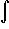#Interactive Real Analysis

Next | Previous | Glossary | Map

## 7.2. Integration Techniques

### Example 7.2.6(b): Applying Integration by Parts

Findx2 cos(x) dx
This time we define
• g'(x) = cos(x) so that g(x) =cos(x) dx = sin(x)
• f(x) = x2 so that f'(x) = 2x
Then G(x) = f(x) g(x) = sin(x) x2 andx2 cos(x) dx = G(b) - G(a) -2x sin(x) dx
Intergration by parts reduced the original problem to finding a slightly simpler integral, but we can not find the second integral immediately. What worked once might work again so let's use integration by parts on the second integral with:
• g'(x) = sin(x) so that g(x) =sin(x) dx = -cos(x)
• f(x) = 2x so that f'(x) = 2
so that G*(x) = -2x cos(x). Therefore2x sin(x) dx = G*(b) - G*(a) + 2cos(x) dx
Taking everything together we have:x2 cos(x) dx = G(b) - G(a) - [G*(b) - G*(a) + 2cos(x) dx ] =
= G(b) - G(a) - G*(b) + G*(a) - 2 (sin(b) - sin(a)) =
= sin(b) b2 - sin(a) a2 + 2b cos(b) - 2a cos(a) -2sin(b) + 2sin(a)
Next | Previous | Glossary | Map